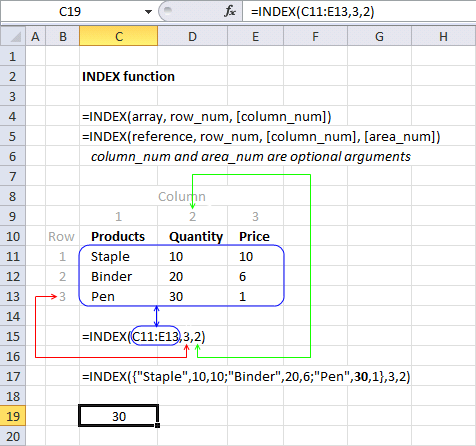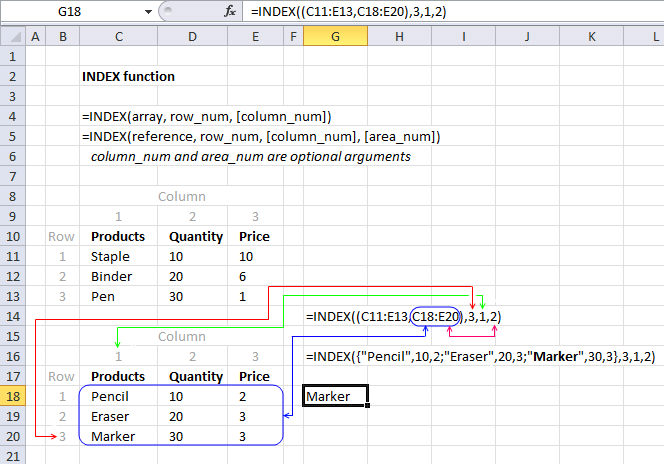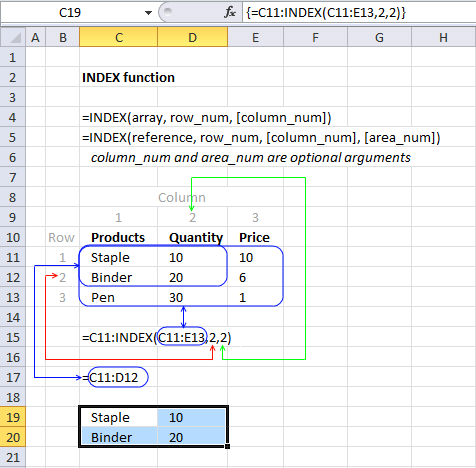Author: Oscar Cronquist Article last updated on April 17, 2018

The INDEX function returns a value from a cell range, you specify which value based on a row and column number.

### Excel Function Syntax

INDEX(array, [row_num], [column_num])

### Arguments

 array or cell reference Required. The cell range you want to get a value from. [row_num] Optional. The relative row number of a specific value you want to get. If omitted the INDEX function returns all values if you enter it as an array formula. [column_num] Optional. The relative column number of a specific value you want to get. If omitted the INDEX function returns all values if you enter it as an array formula. [area_num] Optional. Selects a range in first argument.

### Example 1 - array argument

The first argument in the INDEX function is array. What is an array? An array is a range of cells. To demonstrate in greater detail that an array is an array of values you can convert an array or a cell reference to a static group of values by pressing F9.

Read more about converting cell references or formulas: Replace a formula with its result{"Staple",10,10;"Binder",20,6;"Pen",30,1} each value is separated by a delimiting character. Comma (,) is used to separate columns and semicolon (;) to separate rows.

The english language version of excel uses commas and semicolons, other language versions of excel may use other characters.

### Example 2 - Row_num argument

The second argument in the INDEX function is row_num. It selects the row in an array from which to return a value.Cell range C9:C14 has values separated by a semicolon. The cell range is one dimensional. In this example the value from the second row will be returned.

### Example 3 - column_num argument

The column_num argument selects a column from which to return a value. It is optional if you only have values in a single column, like the example above.Cell range C11:E13 has values separated by commas and semicolons. The cell range  C11:E13 is two dimensional.In this example, the value in the third row and the second column is returned.

### Example 4 - Area_num argument

Area_num selects a range in reference from which to return a value.INDEX(referencerow_num[column_num], [area_num])

INDEX((C11:E13,C18:E20),3,1,2) has two references to two different cell ranges. The area_num selects from which cell reference to return a value. In this example area_num is two and the second cell reference is used. The item in the third row and first column is returned.

### Example 5 - Return a range of valuesThe formula in cell C19:E19 is an array formula. Select cell range C19:E19. Type =INDEX(C11:E13,3,0) in formula bar. Press and hold CTRL + SHIFT. Press Enter. Release all keys.

The example below returns all values from a column.The example below returns all values from a two dimensional cell range.

### Example 6 - Index returns a cell referenceArray formula in cell range C19:D20:

=C11:INDEX(C11:E13,2,2)

INDEX(C11:E13,2,2) returns cell reference D12

C11:D12 returns {"Staple",10;"Binder",20}

### Final note

There are some magic things you can do with the array argument. See this post: No more array formulas?